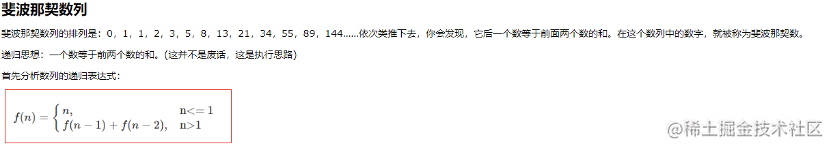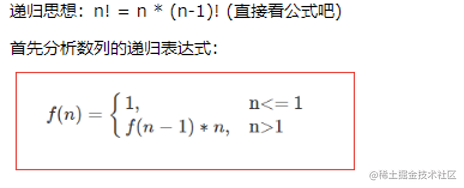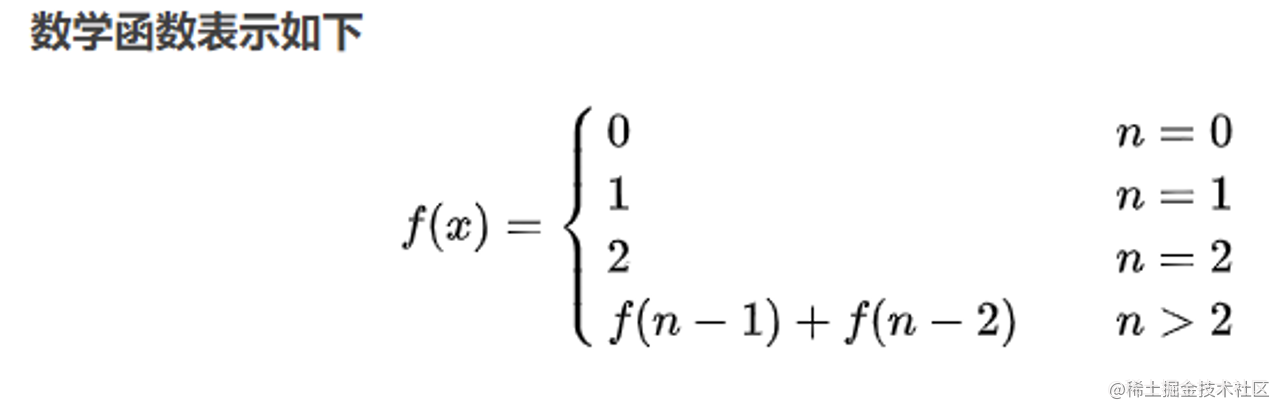# 斐波那契数列由上图总结的规律可知，这样一个规律： f(n) = f(n-2) + f(n-1)； 那么我们很容易就能想到用递归来解决。

• 当 n <=0 时，返回0；
• 当1 <= n <= 2时 返回 1 ；
• 当 n >= 3时，第n项 f(n) = f(n-2) + f(n-1)；
``````function fn(n) {
if (n == 0) {
return 0
} else if (n == 1) {
return 1
} else {
return fn(n - 1) + fn(n - 2)
}}

# 阶乘思路同上一样：初始化第0项和第1项作为已知项； 后面的每一项都是用前面的已知项乘上自己

``````function fn(n) {
if (n<=1){
return 1
}
else {
return fn(n-1)*n
}
}

# 青蛙跳台阶问题

• 如果只有1级台阶，那显然只有一种跳法
• 如果有2级台阶，那么就有2种跳法，一种是分2次跳。每次跳1级，另一种就是一次跳2级
• 如果台阶级数大于2，设为n的话，这时我们把n级台阶时的跳法看成n的函数，记为f(n),第一次跳的时候有2种不同的选择：一是第一次跳一级，此时跳法的数目等于后面剩下的n-1级台阶的跳法数目，即为f(n-1),二是第一次跳二级，此时跳法的数目等于后面剩下的n-2级台阶的跳法数目，即为f(n-2),因此n级台阶的不同跳法的总数为f(n-1)+ f(n-2)，不难看出就是斐波那契数列``````function step(n) {
if (n==0){
return 1
}
if (n==1){
return 1
} if (n==2){
return 2
}
return step(n-1)+step(n-2)
}

# 矩形覆盖问题

• 若最左边竖着放，占了一列,还剩n-1列，剩下为F(n-1)种，
• 若横着放，占了两列，还剩n-2列，剩下为F(n-2)种。仍然是斐波那契数列
``````function step(n) {
if (n==1){
return 1
}
if (n==2){
return 2
}
return step(n-1)+step(n-2)
}